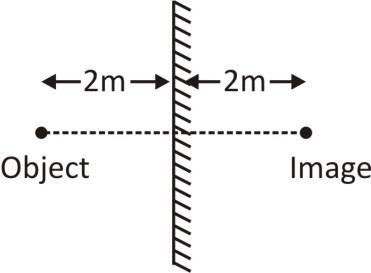QuestionAnswers

# If you take a photograph of your image in a plane mirror. How many metres away should you set your focus if you are 2m in front of the mirror?

Hint: The image formed by a plane mirror is always virtual, upright is at the same distance from the mirror as is the object and is some shape and size as that of the object.

A plane mirror is a mirror with a flat reflective surface. For light rays striking a plane mirror, the angle of reflection equals the angle of incident. The angle of incidence is the angle between the incident rays and the surface normal and the angle of reflection is the angle between the reflected ray and the normal.
A plane mirror makes an image of objects in front of the mirror; these images appear to be behind the plane in which the mirror lies.
So, now we know that the distance of the object from the mirror will be the same as the distance of the image from the mirror.
In this case the object is 2m in front of the mirror.
So, clearly the image will be 2m from the mirror inside it.Now the distance at which you should focus will be equal to distance between object and image.
Total distance = 2m + 2m = 4m

So, the correct answer is 4m.

Note: Here we should know that the focal length of a plane mirror is infinite and its optical power is zero.